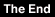## A Discussion on Bezier Curves

by Joel Mathew Hegberg

Bezier curves are named after the French mathematician Pierre Bezier, who developed them for automobile design. What is a Bezier curve? It is the curve which is formed by repeatedly connecting the midpoints of the lines formed by four defining points. "Come again?", you are probably saying. Well, imagine (or draw) four points. Using three lines, you can connect these four points (to form a U shape if the points are positioned carefully). Next, you can draw two more lines connecting the midpoints of the previous 3 line segments. You will now have four inner lines, which you can draw more line segments to connect their midpoints, and so on. This can be repeated many times (infinitely, in fact, which is where Calculus comes into play) and in the end a curve is formed.

Ok, so what? Kinda neat, but how can Bezier curves be used in "real life," like computer programming? Well, assuming computer programming is "real life," Bezier curves are reportedly being used in scalable font technology, since you can define four general points and have a smooth curve be calculated at any size. Since the midpoints can be calculated an infinite number of times, a font made out of bezier curves would be infinitely scalable. Although the word "curve" is used, you can also create straight lines by simply having the four defining points line up in a row.

The equations used to calculate a Bezier curve are third degree equations, meaning they have variables raised to the third power. Let's say our four points are named (x0,y0), (x1,y1), (x2,y2), and (x3,y3). Also, the variable "k" can be used to count from zero to one, so when k=0, the curve is at (x0,y0) and when k=1, the curve is at (x3,y3). (x0,y0) and (x3,y3) are known as the endpoints and (x1,y1) and (x2,y2) are known as "direction" or "control" points. The Bezier curve equations turn out to be:

```x(k)=(1-k)^3*x0+3*k*(1-k)^2*x1+3*k^2*(1-k)*x2+k^3*x3
y(k)=(1-k)^3*y0+3*k*(1-k)^2*y1+3*k^2*(1-k)*y2+k^3*y3```

Wow! Looks a little complex? Don't worry! Your CoCo can handle it! Let's write a small BASIC09 program to handle these equations for us and draw the resulting Bezier curve. To let this program be compatible with the MM/1 (which is what I'm currently using), I shall change window calls such as LINE, down to BYTE arrays since the MM/1 does not have a GFX2 module, but does have the same windowing codes. The parameters for the program will be the four points (x0,y0)(x1,y1)(x2,y2)(x3,y3) and a resolution variable. Since we don't have infinite resolution on our computers (that would be interesting...) we don't have to go through the calculations an infinite number of times! We only need to go through the calculations the number of times necessary to meet our screen resolution. If your are on a 640x200 screen, use 640. Anything higher will not give you a better graph, since you only have 640 dots across your screen. If you choose a number less than 640 (say 100), the computer can draw the curve faster, but it will be less smooth.

Note: BASIC09 takes a very long time to calculate powers (^), so in the program I've changed the power functions into multiplication, since k^2 is the same as k*k, etc.

```PROCEDURE bezier
param x0,y0,x1,y1,x2,y2,x3,y3,res:integer
dim k,r,x,y:real
dim xplot,yplot:integer
dim setdptr(6),linem(6):byte
base 1
setdptr(1)=\$1b \ setdptr(2)=\$40 \ setdptr(5)=0 \ (*SetDPtr window code*)
linem(1)=\$1b \ linem(2)=\$46 \ linem(5)=0 \ (*LineM window code*)
r=1.0/float(res)
k=0.0
setdptr(3)=(x0/256) \ setdptr(4)=land(x0,255)
setdptr(6)=land(y0,255)
put #1,setdptr \ (*Set draw pointer on stdout*)
while (k <=1.0) do
x=(1-k)*(1-k)*(1-k)*x0+3*k*(1-k)*(1-k)*x1+3*k*k*(1-k)*x2+k*k*k*x3
y=(1-k)*(1-k)*(1-k)*y0+3*k*(1-k)*(1-k)*y1+3*k*k*(1-k)*y2+k*k*k*y3
xplot=int(x)
yplot=int(y)
linem(3)=(xplot/256) \ linem(4)=land(xplot,255)
linem(6)=land(yplot,255)
put #1,linem \ (*Draw line on stdout*)
k=k+r
endwhile
end
```

Enter this into BASIC09 (or BASIC on an MM/1) and PACK it to your CMDS directory as instructed in your BASIC09 manual. To use the program, you must be on a graphics screen. On the MM/1, this is no problem since you are always on a graphics screen. On the CoCo, if you want to set up a graphics screen on, say, window 5, use:

```wcreate /w5 -s=7 0 0 80 24 1 0 4
shell i=/w5&```

Then CLEAR over to the newly created graphics window. Now, run the program using the format:

```bezier(x0,y0,x1,y1,x2,y2,x3,y3,res)   ; for the CoCo or
bezier x0 y0 x1 y1 x2 y2 x3 y3 res    ; for the MM/1.```

Try some of the following for some interesting curves:

```bezier(50,50,20,80,100,150,60,10,100)
bezier(50,190,20,80,200,150,60,10,100)
bezier(600,100,340,20,310,190,610,55,100)
bezier(320,100,0,200,640,200,320,100,100)  (Hershey's Kiss)
bezier(320,50,320,200,200,200,180,140,100)  (The letter "J")```